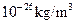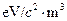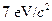Chapter 34, Problem 28PE

Chapter
Section
Textbook Problem

The critical mass density needed to just halt the expansion of the universe is approximately(a) Convert this to(b) Find the number of neutrinos per cubic meter needed to close the universe if their average mass isand they have negligible kinetic energies.

To determine

(a)

The critical mass density in terms of eV/c2m3.

Explanation

Given:

Critical mass density, ρ=1026kg/m3

Calculation:

Consider the conversion factor to convert the critical mass density in eV/c2m3.

ρ=1026×9×1016×(1 1

To determine

(b)

The number of neutrinos per cubic meter.

Still sussing out bartleby?

Check out a sample textbook solution.

See a sample solution

The Solution to Your Study Problems

Bartleby provides explanations to thousands of textbook problems written by our experts, many with advanced degrees!

Get Started